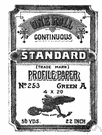# vector product

Also found in: Thesaurus, Encyclopedia, Wikipedia.
Related to vector product: vector multiplication

## vector product

n.
A vector c, depending on two other vectors a and b, whose magnitude is the product of the magnitude of a, the magnitude of b, and the sine of the angle between a and b. Its direction is perpendicular to the plane through a and b and oriented so that a right-handed rotation about it carries a into b through an angle not greater than 180°. The notation for c is c = a × b. Also called cross product.

## vector product

n
(Mathematics) the product of two vectors that is a pseudovector, whose magnitude is the product of the magnitudes of the given vectors and the sine of the angle between them. Its axis is perpendicular to the plane of the given vectors. Written: A × B or AB. Also called: cross product Compare scalar product
Collins English Dictionary – Complete and Unabridged, 12th Edition 2014 © HarperCollins Publishers 1991, 1994, 1998, 2000, 2003, 2006, 2007, 2009, 2011, 2014

## cross′ prod`uct

n.
a vector perpendicular to two given vectors and having magnitude equal to the product of the magnitudes of the two vectors multiplied by the sine of the angle between them. Also called vector product.
[1925–30]
ThesaurusAntonymsRelated WordsSynonymsLegend:
 Noun 1vector product - a vector that is the product of two other vectorscross productvector - a variable quantity that can be resolved into components
Based on WordNet 3.0, Farlex clipart collection. © 2003-2012 Princeton University, Farlex Inc.
Translations
Kreuzprodukt
References in periodicals archive ?
DWD introduces a first configuration of the new system based on NEC's unique vector product NEC SX-Aurora TSUBASA with identical performance compared to their current system by the end of this year.
Oxford BioMedica is a world leader in lentiviral vector product development and manufacturing, and will be the clinical and commercial supplier of AXO-Lenti-PD.
In all these definitions the angular momentum is defined as a vector product containing the position coordinate.
To explain, first it is beneficial to demonstrate full O([N.sup.2.sub.b][M.sup.2.sub.b]) matrix vector product which explicitly loads each matrix element,
The orientation of the magnetic induction vector is perpendicular to plane defined by vectors RS and ST, meaning it is equal to orientation of their vector product. Hence, it is necessary to determine cosines of the angles formed by aforementioned product and coordinate axes.
Additionally, the multilevel fast multipole algorithm (MLFMA) [15-23] is utilized to accelerate the computations of matrix vector product. Numerical examples have demonstrated the validity and efficiency of the proposed scheme.
For any two vectors x = ([x.sub.1],[x.sub.2],[x.sub.3]) and y = ([y.sub.1],[y.sub.2],[y.sub.3]) of [E.sup.3.sub.1], the inner product is the real number < x, y >= [x.sub.1][y.sub.1] + [x.sub.2][y.sub.2] - [x.sub.3][y.sub.3] and the vector product is defined by x x y = (([x.sub.2][y.sub.3] - [x.sub.3][y.sub.2]), ([x.sub.3][y.sub.1] - [x.sub.1][y.sub.3]), ([x.sub.1][y.sub.2] - [x.sub.2][y.sub.1])).
If the matrix that represents the vector product is defined (3), it is possible to show the relation (2) as a product of matrices (4).
Drift, and the observable ground track on the scope, is a vector product of TAS and crosswind component.
Neutrons propagate along an axis y and an axis x is the direction of the vector product [k X I].

Site: Follow: Share:
Open / Close